# Pointing the CBI.

The following material describes how geocentric apparent ra,dec (and distance for nearby objects) can be converted to instrument azimuth (az), elevation (el), parallactic angle (pa) and associated tracking rates. This involves finding where an ideal telescope at the longitude and latitude of the CBI would need to point, and then incorporating the effects of axis tilts to determine the equivalent instrumental az,el,pa.

## Gather site-specific parameters

For a given site we need the following site-dependent parameters:
•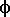- The geodetic latitude of the site.
•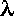- The geodetic longitude of the site.
• Rc - The distance from the center of the Earth (m).
• Rr - The radial distance from the rotation axis of the Earth (m).
The geodetic latitude, longitude and height above sea level will be determined from GPS measurements. The Earth isn't quite spherical, so one of the standard reference spheroids must be chosen to compute the the dimensions of the Earth. The CBI will use the spheroid that is coded in the slalib `slaGeoc()` function. When passed the geodetic latitude and height above sea-level, this returns the radial distance from the rotation axis, Rr, and the distance from the equatorial plane, denoted by `z`. The distance from the center of the Earth is then given by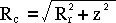The altitude returned by GPS probably won't be accurate enough for tracking the moon to arcsecond precision. In particular it may (?) be referenced to a different reference spheroid than `slaGeoc()`. If this ever becomes an issue, the altitude will have to be determined more accurately, perhaps by adjusting it interactively to produce stationary fringes while tracking the limb of the moon. For other, more distant sources, the accuracy of the GPS altitude should be more than sufficient.

## Spherical coordinate systems

Where possible, spherical trigonometry will be used to compute positions and corrections on the celestial sphere. The following diagram shows the chosen form of the celestial sphere.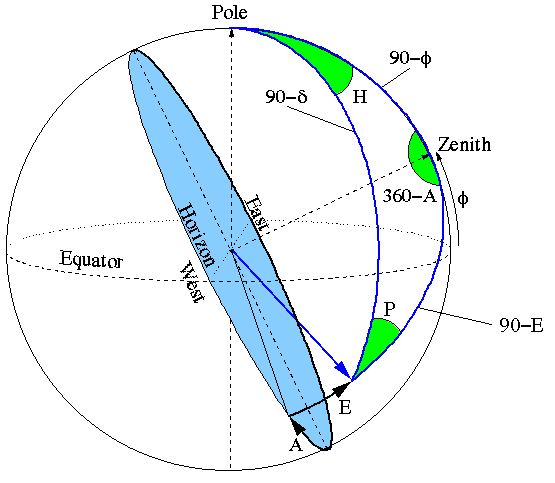The symbols shown in this diagram are used throughout this document. They are defined as:

•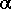- Geocentric apparent Right Ascension.
•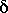- Geocentric apparent Declination.
•- Geodetic lattitude.
• H - Hour Angle (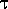-).
• A - Azimuth. N=0o E=90o S=180o W=270o
• E - Elevation above the local horizon.
• P - Parallactic Angle.
•- Local apparent sidereal time (radians).
• T - UT1.
Application of the sine formula of spherical trigonometery to the displayed triangle yields: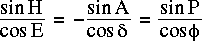Similarly the cosine formula of spherical trigonometery yields: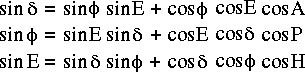and the analogue to the cosine formula yields: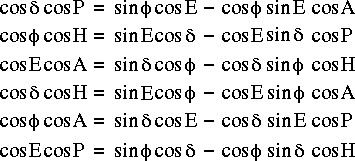The above equations can be combined to find the horizon coordinates that correspond to given equatorial coordinates. The resulting equations are: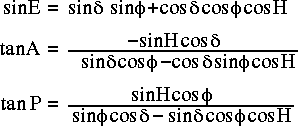For the CBI we also need to know the rates at which A,E and P evolve per second of UT. For a sidereal source this is determined solely by the rotation of the earth, so the rates are related to the steady increase in the hour angle of the source. The rates due to precession are insignificant. For a nearby source an additional contribution comes from the apparent proper motion of the source. This appears as a steady change inand. For the computation of tracking rates the proper motion terms can be ignored for all standard astronomical sources except the moon. However it is possible that ephemerides could be used to specify unusual tracks, such as raster scans of the sky, so in the CBI, the proper motions of all sources that are implemented as ephemerides will be incorporated into the tracking rates. The proper motions of non-ephemeris objects will be ignored.

The tracking rates can be found by differentiating the above equations wrt hour angle, then multiplying the results by the sidereal rate.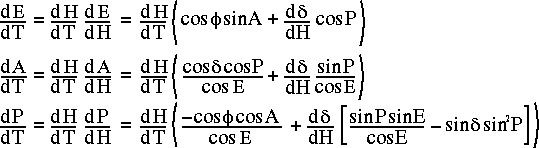where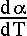and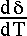represent the proper motion of the source (if any), and: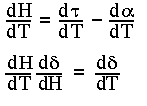Note that d/dT is the ratio of the rotational period of the Earth to the length of the UT1 day. According to the explanatory supplement of the Astronomical Almanac, when the ratio of mean sidereal time to UT1, 1.002737909350795, is combined with the precession rate of right ascension, the rotation rate of the Earth is reasonably aproximated as 1.002737811906 rotations per UT1-day. In order to compute tracking rates in radians per UT1 second, the appropriate value for d/dT is thus.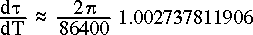## Conversions from geocentric to topocentric coordinates.

When applied to geocentric equatorial coordinates, the preceding equations return horizon coordinates for an observer at the center of the Earth. The corrections in the following sections move the observer to the appropriate point on the surface of the Earth and correct for distortions caused by inevitable inperfections in the alignment of the physical axes of the telescope.

## Account for horizontal parallax

The line of sight of the CBI to a nearby object is inclined to the line that connects the center of the Earth to the object. The geometry is shown below.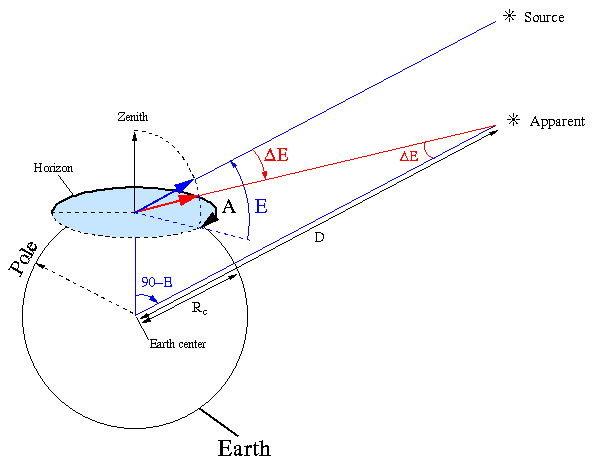Application of the sine rule to the above triangle reveals,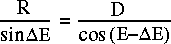Solving this for the angle of parallax results in,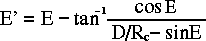This correction will be applied to solar system objects only.

## Account for atmospheric refraction

Atmospheric refraction causes objects to appear at slightly higher elevations than they otherwise would. The geometry is illustrated in the following diagram.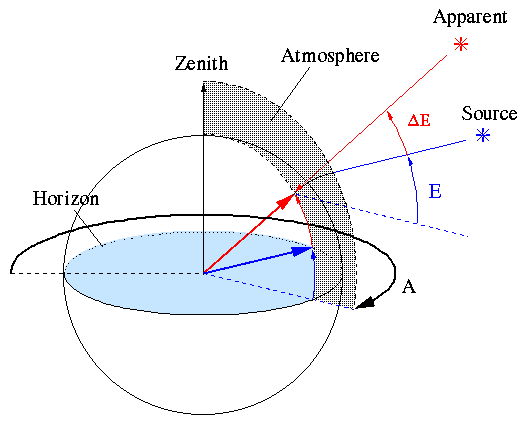The CBI weather station will provide real-time measurements of the local temperature and relative humidity. This will be used to estimate conditions in the higher parts of the atmosphere and thus derive an estimate of the elevation offset due to refraction. Rather than devising an empirical model of the atmosphere, the CBI will, at least initially, adopt the Starlink `slaRefro()` function in Patrick Wallace's slalib library. This was originally developed with optical pointing in mind, but it was subsequently retrofitted for radio wavelengths. It remains to be seen whether this will be adequate for the CBI. If not, an empirical model will have to be devised. Unfortunately the conditions in Pasadena are radically different from those on Chajnantor, so initial testing at Caltech won't necessarily resolve this issue.

Given information about local atmospheric conditions `slaRefro()` returns two coefficients, `A` and `B`. These relate the vacuum elevation to the observed refraction via the following equation.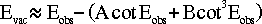We need an equation that goes the other way, from the vacuum elevation to the observed elevation. Unfortunately the above equation is transcendental, so in Starlink User Note 67, Pat Wallace suggests the following approximation.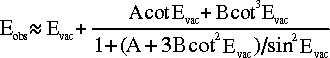This can be expanded to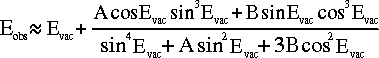## Account for diurnal aberration.

In a moving frame with a component of its velocity perpendicular to the line of sight to a light source, an observer sees the light coming from a direction slightly ahead of its actual position. The geometry of the situation is illustrated below.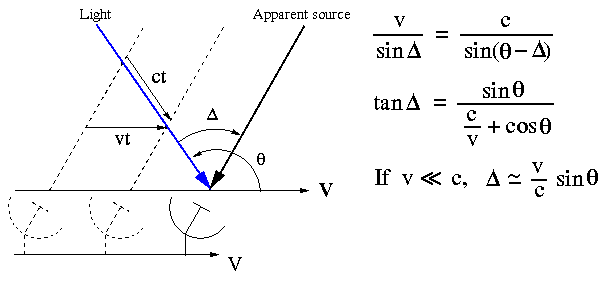Because of this effect, the rotational velocity of the surface of the earth results in a small diurnal shift in the apparent positions of sources. The maximum aberration at the latitude of the CBI is about 0.3 arcseconds. Note that the much larger effect caused by the motion of the Earth around the Sun is assumed to have been taken care of during the precession calculations used to find the apparent Right Ascension and Declination of the source.

The tangential rotational velocity at the surface of the Earth is given by,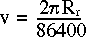Where Rr was defined earlier to be the radial distance of the CBI from the center of the Earth, and 86400 is the number of seconds in a day.

To determine the adjusted azimuth and elevation, the following diagram shows a unit vector pointing at the source from the center of the celestial sphere, overlaid with a rectangular coordinate system that is aligned with the compass points and the zenith. A second unit vector illustrates the effect of diurnal aberration, and the accompanying equations show how to calculate its direction.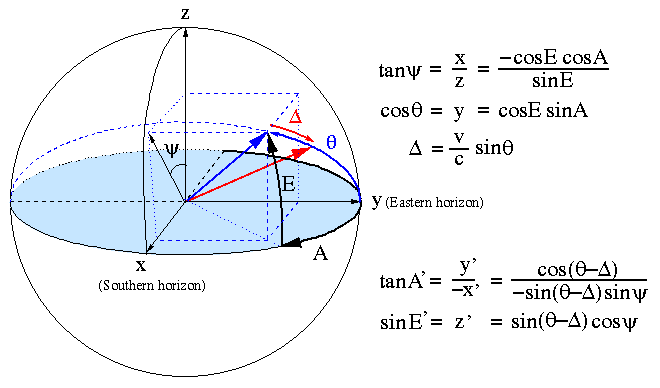## Account for telescope flexure.

In a traditional radio telescope a single elevation axis supports a dish and a large counterweight. Gravity has the effect of causing some sag either side of this axis and thus causes the elevation to be lower than requested. The CBI should be largely immune to this effect because the platform on which its dishes are mounted is supported at two places, as illustrated in the following diagram.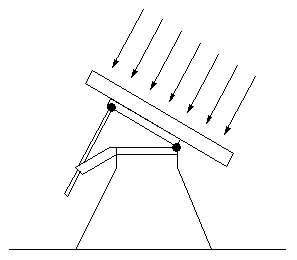Nonetheless, there will be some flexure in the mount itself and in the azimuth bearing. This should be a function of elevation. Traditionally one assumes that flexure is proportional to the torque applied by gravity to a hypothetical telescope tube. This can be corrected using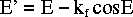where kf is an empirical constant to be fitted for. It remains to be seen whether this will be appropriate or necessary for the CBI.

## Accounting for the tilt of the Azimuth axis.

The azimuth axis of the telescope doesn't lie exactly along the line dictated by the geodetic longitude and latitude reported by the GPS receiver. There are two reasons for this.
1. Whereas the azimuth axis is aligned as closely as possible to the local direction of gravity, local gravity is unlikely to exactly match that predicted by the spheroidal model of the Earth that is used by GPS.
2. Even if we managed somehow to level the azimuth platform to the required degree of accuracy, irregularities in the azimuth bearing and the varying center of gravity of our non-counterweighted deck platform would quickly destroy this alignment.
The result is a tilt of the azimuth axis, parameterized by its size,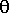and its azimuth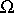. The following diagram depicts this tilt, along with the effect it has on three example source positions. Note that the known parameters are written in blue, and the unknowns in red.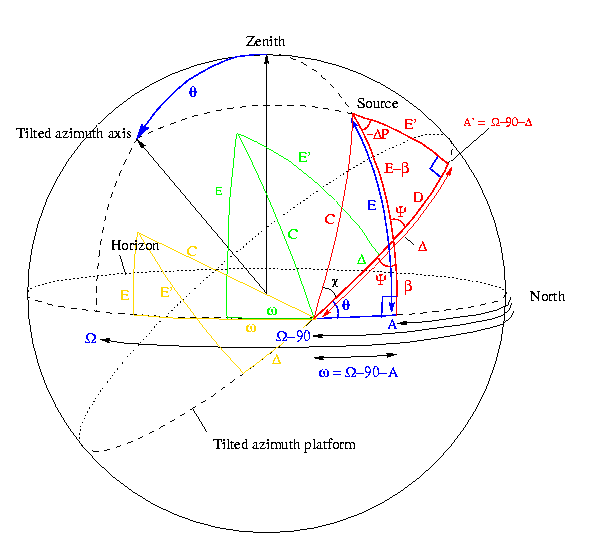From this diagram, given a topocentric source position (A,E,P) we need to find the new azimuth,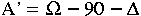, the new elevation, E' and the new paralactic angle, P'=P+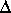P, that correspond to the tilted coordinate system of the telescope.

By applying the sine rule of spherical trigonometry to the lower left and rightmost triangles, and the 4-parts formula to the lower left triangle, one finds that: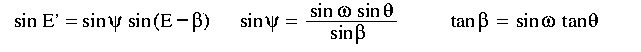from which one can solve for E'.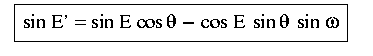By applying the four-parts formula separately to the left and right triangles, one obtains two equations for tan(C), which in turn can be solved for the following equation for tan: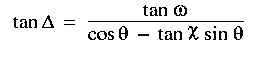where the unknown, tan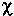, is given by applying the 4-parts formula again to a different combination of parameters of the left triangle, resulting in: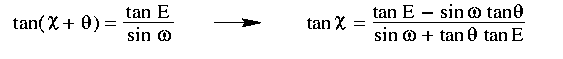Substituting this into the previous equation gives: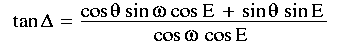This can then be used to compute the new azimuth,,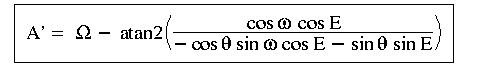By applying the 4-parts formula again to two different sets of parameters of the rightmost triangle, plus the 4-parts formula to the lower left triangle, one can also obtain: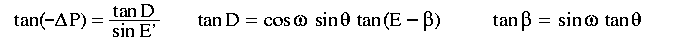Using these equations to solve forP, gives: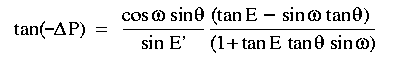which after having its denominator and numerator multiplied by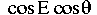results in: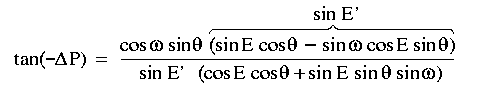Thus the apparent parallactic angle of the source is: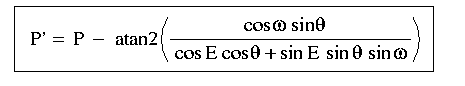As mentioned above there are two main parts to the tilt.

1. The discrepancy between the local direction of gravity and the direction assumed by the GPS spheroid, denoted by (A,A). This is a fitted component of the pointing model.
2. The variable tilt of the azimuth platform with respect to the local vertical. In the case of the CBI, this is measured on-line by two tilt meters situated on the azimuth platform. The following diagram shows their orientation. Note that the two directions that the tilt meters measure, rotate with the azimuth platform.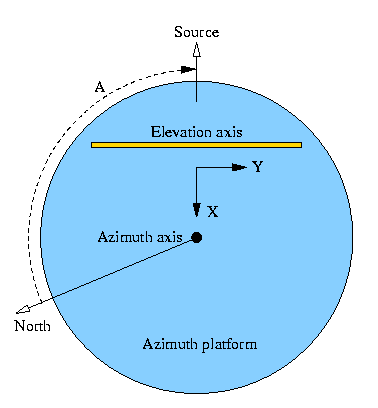To help clarify this diagram, note that to first order, tilting the front of the azimuth platform upwards corresponds to a positive tilt reading on the X-axis tilt meter, so the telescope ends up pointing at a higher elevation than expected.

In order to use the tilt meter readings to correct for the variable part of the tilt we first have to convert from the two orthogonal X,Y readings to their polar equivalents,T andT. The following diagram illustrates this.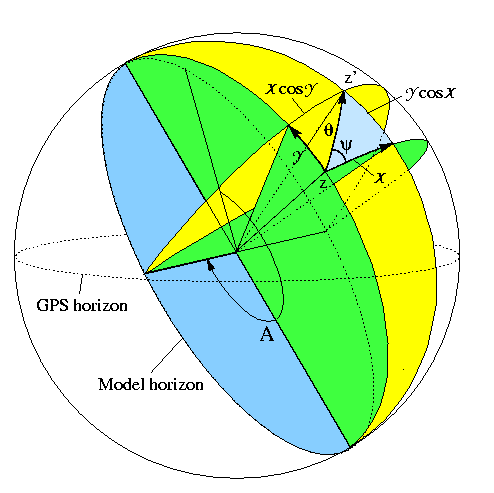Applying the four parts and cosine formulae to the highlighted triangle gives the following equations: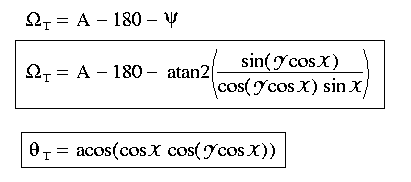The composite tilt of the azimuth axis is thus corrected by applying the tilt correction equations first to the model-fitted tilt (A,A), then to the variable tilt (T,T), computed from the latest tilt meter readings.

## Account for the non-perpendicularity of the Elevation axis.

The elevation axis is supposed to lie in the plane of the azimuth ring, along the line joining A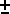90 degrees. In practice there will be a small angle between this axis and the actual elevation axis. The component of the tilt that lies within the plane of the azimuth is indistinguishable from an azimuth encoder offset, and is dealt with later. The remaining component of the tilt manifests as a rotation about the radial azimuth vector, and will be denoted by the clockwise angleE, as seen from azimuth bearing. In the following diagram E' and A' are the azimuth and elevation that, when modified by the elevation-axis tilt, result in the requested azimuth and elevation.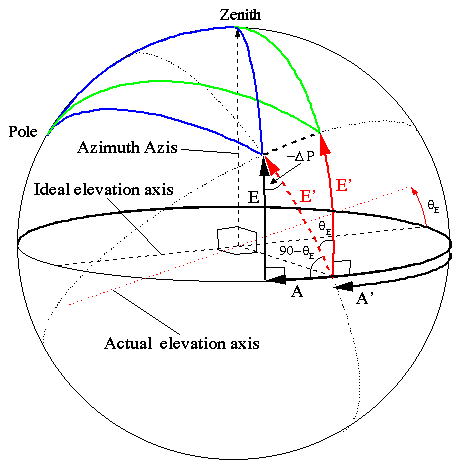By applying, the spherical trigonometry sine, analogue to the cosine, and a variant of the cosine formula to the bottom left triangle, one obtains the following equations.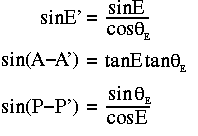## Account for collimation errors

The 13 radio dishes and a small optical telescope are mounted on a rotating platform, hereafter called the "deck". This rotates to keep the field of view from appearing to rotate. The optical axes of the dishes and the optical telescope won't exactly parallel the rotation axis of the deck, so unless corrective action is taken, a point source will appear to describe a circle on the sky as the deck rotates.

The CBI will need two separate collimation models.

1. When the telescope is observing radio sources, a collimation model that accounts for the tilt of the deck and any common tilt in the dishes will be used. The collimation errors of individual dishes can't be addressed by a single collimation model, so they will have to be corrected during post-processing.
2. When the optical telescope is being used to measure the rest of the pointing model, a collimation model that counters both the tilt of the deck and the misalignment of the optical telescope will be used.
In both cases the error can be corrected by adjusting the demanded azimuth and elevation in accordance with the rotation angle of the deck.

The position angle of the deck will be denoted by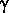. This increases as the deck rotates clockwise around the direction of the elevation vector and is here defined to point in the direction of increasing elevation whenis zero. While tracking a source, the position angle of the deck will be rotated to keep the field of view from rotating. The deck angle will thus be given by,=o + P.

Whereo is a user selected deck-angle offset and P is the corrected parallactic angle of the source. The collimation tilt will be defined by a position angle offset,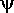c, and a magnitudec. The following diagram indicates the azimuth and elevation adjustments required to point at a source at the target azimuth and elevation.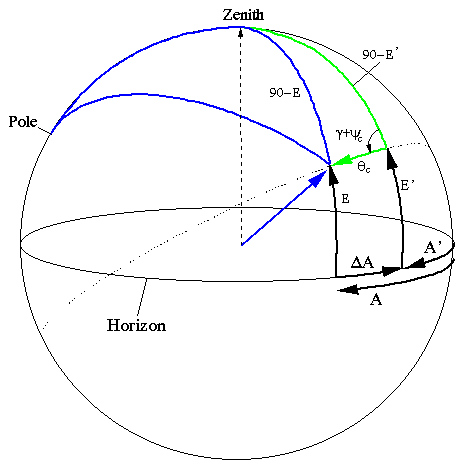The equations that relate the adjusted azimuth and elevation to the target elevation and azimuth are given by applying, respectively, the sine and cosine spherical trigonometry formulae to the green triangle.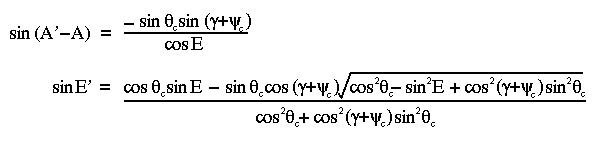Note that exploiting small-angle expectations to approximate cos2c to 1, and sin2c to 0 orc2, could result in significant errors, particularly near the zenith. For example, if+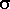=90o, E=80o andc=0.1o, then the returned elevation will be wrong by almost 2 seconds of arc.

Also note that the apparent parallactic angle is not effected by collimation errors.

## Compute equivalent Hour Angle and Declination

Having deduced the azimuth, elevation to be demanded, we still need to determine the the axis drive rates. The equations for the drive rates, in radians per second, are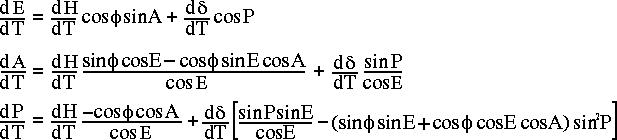Note that terms involvinghave been replaced by equations involving `sin` and `cos` of the corrected A,E and P.

It remains to convert the positions and rates to encoder units. This involves multiplying by a fixed conversion factor and adding measured encoder offsets.

## The components of the pointing model

The pointing model, described above, contains 9 terms that must be deduced from observations of calibration sources. The 9 terms are,

 kf The constant of flexure (degree per cosine of elevation).HA The tilt of the azimuth axis in the direction of increasing hour angle.A The tilt of the azimuth axis in the direction of increasing latitude.E The tilt of the elevation axis perpendicular to the azimuth ring, measured clockwise around the direction of the azimuth vector. It makes sense to cache the values of sinE and cosE.c The magnitude of the collimation error. It makes sense to cache the values of sinc and cosc.c The deck angle at which the collimation error is directed radially outward. Eo The elevation encoder count at zero elevation. Ao The azimuth encoder count at zero azimuth. Po The deck encoder count that aligns the reference edge of the triangular platform to be horizontal. The reference edge should be chosen such that Po is close to zero.

# Tracking offsets

While tracking a source using the above pointing model, it is useful to be able to offset the telescope from the target position. For example:
1. While measuring the parameters that go into the pointing model, small azimuth and elevation offsets are entered manually to compensate for errors in the model and bring the wandering star back into the center of the field. After making such adjustments on a lot of stars around the sky, the pointing model parameters can then be measured off-line by fitting the pointing model to the adjusted positions. These offsets are estimated from looking at the television output of a CCD camera that is mounted on the back of a small optical telescope that is rigidly attached to the moving deck platform. There thus needs to be a command that takes x/y distances on the TV and converts them to telescope azimuth and elevation offsets, irrespective of the rotation angle of the deck platform, and any mirroring effects in the telescope or the CCD camera.

2. To measure the beam profile of the antennas one samples the beam pattern with a strong unresolved radio source sequentially placed at each of the vertices of a rectangular grid of positions around the pointing center. The coordinate system of the grid must be independent of the location of the field center, so it can not be implemented using fixed azimuth/elevation or Right-Ascension/Declination offsets. Instead we use a spherical coordinate system centered on the field center. To orient this system it is convenient to use the great circle that joins the field center and the zenith as one of two orthogonal great circles. This is refered to as the Y axis, and it increases from the field center to the pole. The X axis increases in the same sense as the azimuth, but is only parallel to it when the telescope is pointing at zero elevation.

The following diagram illustrates the relationship between the X,Y offsets and the resulting azimuth and elevation corrections.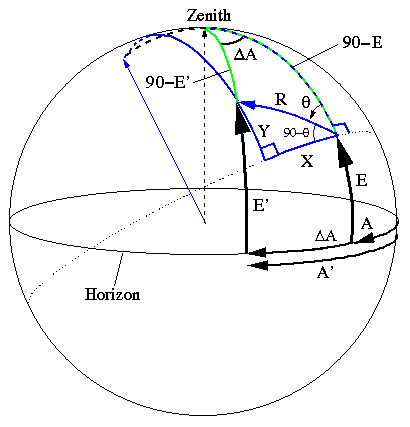The corresponding equations are: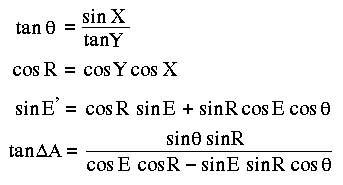Note that since the offset depends on the azimuth and elevation of the pointing center, which changes as the source moves across the sky, this offset has to be recomputed every servo cycle.

3. While mosaicing multiple fields around a reference field, the fields must be fixed on the sky. This is easily accomplished by using a grid of fixed Right-Ascension and Declination offsets. To give the grid approximately equal spacings in the two directions, it is sufficient to scale the Right-Ascension offsets by the cosine of the Declination of the corresponding grid point.

Martin Shepherd (Last updated 29-Mar-2001)